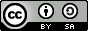# NandakumarContent Network

## Simple Interest Calculator in Python

By | |Here is a very simple Python code snippet which can be used as a simple interest calculator.

```print("SIMPLE INTEREST CALCULATION\n") print("Enter the following details.") p = input("Amount: ") n = input("No. of Years: ") r = input("Rate of Interest: ") i = p*n*r/100 a = p + i print("\nThe interest is " + str(i)) print("The total amount is " + str(p + i)) ```

Run online: Copy the above code and Click here to visit the page (by tutorialspoint.com) where you can paste and run it online.

The code is self-explanatory. However, let's make some points clear:

• '\n' used in print statements simply means a newline.
• The str() function is used to convert numbers to string (text) so that they can be displayed using the print() function.

While saving the code as an executable script (i.e., a text file with .py extension and executable permission) in GNU/Linux, please don't forget to add this line at the very beginning of the code: ```#!/usr/bin/env python ```

Keywords (click to browse): simple-interest simple-interest-calculator interest python programming scripting computer technology

Simple Interest Calculator in Python | Nandakumar Content Network